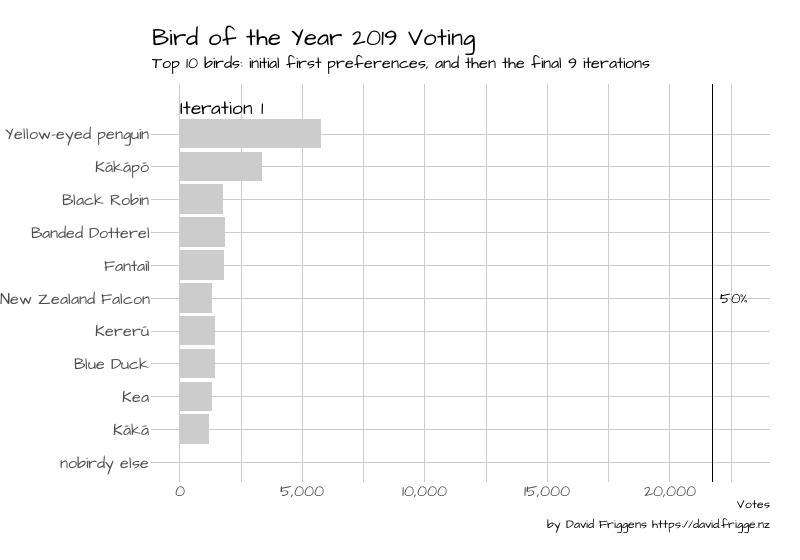# The Yellow-eyed Penguin and the Green-eyed Parrot: Animating voting results

New Zealand birds and their human puppets have been fighting it out for the coveted Bird of the Year title for the 15th time. Here we animate the STV voting using R’s `gganimate` package.

David Friggens
2019-11-16

If you’ve been anywhere near New Zealand Twitter in the past month you will be well aware of the Bird of the Year competition. If not, feel free to read up on Wikipedia. The good folks at Dragonfly Data Science and Forest and Bird have made the voting data available.

There is interesting analysis to be done on the voters and preferences, which I will leave to others, as I’m more immediately interested in the counting. The election allowed five preferences to be chosen, with Single Transferable Vote (STV) used for the count.

This is very similar to the local election STV animations I’ve recently done, so thought I’d knock up something similar. The full counting would be a bit infeasible as there were 85 birds, and it took 84 iterations to decide the winner! So I’ve limited to the top 10 birds, showing the initial first preferences in Iteration 1, and then the final 9 iterations.At first glance it seems laughable that there are so many eliminated ballots by the end. But realising that there are 85 birds in play and you can only rank five, it’s actually quite impressive that half of the voters chose at least one of the top two.

## Code

Here’s the code to produce the animation. Note that I’ve only seen the ballot data, not any information about the official voting details, so the counting here using the `STV` R package may not be exactly the same. It should be close enough though.

This is much simpler that the other ones I’ve done — there’s just one step per iteration. It was fairly straightforward given that experience, though I thought I’d be able to use `transition_states`; this caused some weird behaviour though so I switched to `transition_components`.

``````
library(magrittr)
library(dplyr)
library(forcats)
library(tidyr)
library(STV)
library(ggplot2)
library(gganimate)
library(hrbrthemes)
library(showtext)

showtext_auto()

# one row per ballot, one column per preference
col_types = "___ccccc")

# one row per ballot, one column per bird
bind_rows(
raw_votes %>% select(bird = vote_1) %>% mutate(pref = 1L, id = row_number()),
raw_votes %>% select(bird = vote_2) %>% mutate(pref = 2L, id = row_number()),
raw_votes %>% select(bird = vote_3) %>% mutate(pref = 3L, id = row_number()),
raw_votes %>% select(bird = vote_4) %>% mutate(pref = 4L, id = row_number()),
raw_votes %>% select(bird = vote_5) %>% mutate(pref = 5L, id = row_number())
) %>%
drop_na() %>%
select(-id)

# wait a little while for this one
election <-
stv(formatted_votes %>% as.data.frame(),  # stv hates tibbles
seats = 1) %>%
extract2("details") %>%
mutate(ntv = num_ballots - ballots,
iteration = row_number()) %>%
as_tibble()

# I'm having issues with utf8 on Windows, hence the numbers
data_to_plot <-
election %>%
select(iteration, quota, ntv,
94, # Yellow-eyed penguin
42, # Kākāpō
22, # Black Robin
14, # Banded Dotterel
32, # Fantail
55, # New Zealand Falcon
44, # Kererū
25, # Blue Duck
43, # Kea
41  # Kākā
) %>%
filter(iteration == 1 | iteration >= 76)

quotas <-
data_to_plot %>%
select(iteration,
quota,
ntv) %>%
mutate(iteration_label = paste("Iteration", iteration))

data_to_plot %>%
select(-quota, -ntv) %>%
bind_rows(
quotas %>%
transmute(iteration,
Bird = "nobirdy else",
) %>%
mutate(Bird = Bird %>% fct_inorder() %>% fct_rev(),
bar_colour = case_when(Bird == "nobirdy else" ~ "#333333",
Bird == "Yellow-eyed penguin" & iteration == max(iteration) ~ "yellow",
TRUE ~ "gray80")) %>%
arrange(iteration)

num_iterations <- votes %>% pull(iteration) %>% max()
num_iterations_shown <- votes %>% distinct(iteration) %>% nrow()
num_birds <- votes %>% distinct(Bird) %>% nrow()

# to handle transition_components
timeframes <-
quotas %>%
select(iteration) %>%
mutate(timeframe = row_number())
quotas %<>% inner_join(timeframes)

bird_plot <-
ggplot() +
theme_ipsum(base_size = 12,
base_family = DEFAULT_FONT) +
# draw the bars
aes(x = Bird, y = Votes, group = Bird, fill = bar_colour)) +
scale_y_continuous(labels = scales::comma) +
scale_fill_identity() +
coord_flip() +
# label winner
geom_text(data = votes %>% filter(iteration == num_iterations,
Bird == "Yellow-eyed penguin"),
aes(x = Bird, group = Bird),
y = 500,
label = "WINNER!",
family = DEFAULT_FONT,
color = "black",
hjust = 0
) +
# draw the quota line
geom_hline(data = quotas, aes(yintercept = quota)) +
geom_text(data = quotas,
x = (num_birds + 1) / 2,
aes(y = quota),
label = "50%",
nudge_y = 300,
family = DEFAULT_FONT,
color = "black",
hjust = 0) +
expand_limits(x = num_birds + 1.5) +
geom_text(data = quotas,
aes(label = iteration_label),
x = num_birds + 0.8, y = 0,
size = 5, fontface = "bold", hjust = 0,
family = DEFAULT_FONT) +
# animation controls
transition_components(timeframe) +
ease_aes("cubic-in-out") +
# labels
labs(x = NULL,
title = "Bird of the Year 2019 Voting",
subtitle = "Top 10 birds: initial first preferences, and then the final 9 iterations",
caption = "by David Friggens https://david.frigge.nz")

bird_gif <-
animate(bird_plot,
fps = 10,
duration = num_iterations_shown + 6,
width = 800, height = 560,
start_pause = 10,
end_pause = 10)

anim_save("images/boty2019.gif", animation = bird_gif)``````